# Recursion

## Introduction

Recursion is:

• A way of thinking about problems.
• A method for solving problems.
• Related to mathematical induction.
A method is recursive if it can call itself; either directly:
```void f() {
... f() ...
}
```
or indirectly:
```void f() {
... g() ...
}

void g() {
... f() ...
}
```

You might wonder about the following issues:

Q: Does using recursion usually make your code faster?
A: No.

Q: Does using recursion usually use less memory?
A: No.

Q: Then why use recursion?
A: It sometimes makes your code much simpler!

One way to think about recursion:

• When a recursive call is made, the method clones itself, making new copies of:
• the code
• the local variables (with their initial values),
• the parameters
• Each copy of the code includes a marker indicating the current position. When a recursive call is made, the marker in the old copy of the code is just after the call; the marker in the "cloned" copy is at the beginning of the method.
• When the method returns, that clone goes away, but the previous ones are still there, and know what to execute next because their current position in the code was saved (indicated by the marker).

Here's an example of a simple recursive method:

```   void printInt( int k ) {
1.     if (k == 0) {
2.         return;
3.     }
4.     System.out.println( k );
5.     printInt( k - 1 );
6.     System.out.println("all done");
}
```
If the call printInt(2) is made, three "clones" are created, as illustrated below: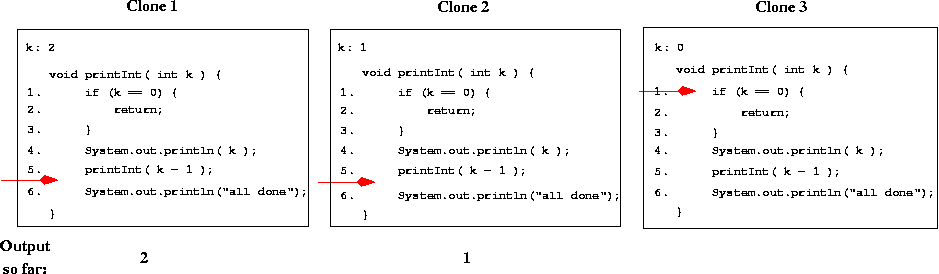The original call causes 2 to be output, and then a recursive call is made, creating a clone with k == 1. That clone executes line 1: the if condition is false; line 4: prints 1; and line 5: makes another recursive call, creating a clone with k == 0. That clone just returns (goes away) because the "if" condition is true. The previous clone executes line 6 (the line after its "marker") then returns, and similarly for the original clone.

Now let's think about what we have to do to make sure that recursive methods work correctly. First, consider the following recursive method:

```void badPrint( int k ) {
System.out.println( k );
}
```
Note that a runtime error will occur when the call badPrint(2) is made (in particular, an error message like "java.lang.StackOverflowError" will be printed, and the program will stop). This is because there is no code that prevents the recursive call from being made again and again and .... and eventually the program runs out of memory (to store all the clones). This is an example of an infinite recursion. It inspires:

*** RECURSION RULE #1 ***

Every recursive method must have a base case -- a condition under which no recursive call is made -- to prevent infinite recursion.

Here's another example; this version does have a base case, but the call badPrint2(2) will still cause an infinite recursion:

```void badPrint2( int k ) {
if (k < 0) return;
System.out.println(k);
}
```
This inspires:
*** RECURSION RULE #2 ***

Every recursive method must make progress toward the base case to prevent infinite recursion.

TEST YOURSELF #1

Consider the method printInt, repeated below.

```void printInt( int k ) {
if (k == 0) return;
System.out.println( k );
printInt( k - 1 );
}
```
Does it obey recursion rules 1 and 2? Are there calls that will lead to an infinite recursion? If yes, how could it be fixed?

## How Recursion Really Works

This is how method calls (recursive and non-recursive) really work:

• At runtime, a stack of activation records (ARs) is maintained: one AR for each active method, where "active" means: has been called, has not yet returned.
• Each AR includes space for:
• the method's parameters,
• the method's local variables,
• the return address -- where (in the code) to start executing after the method returns.
• When a method is called, its AR is pushed onto the stack. The return address in that AR is the place in the code just after the call (so the return address is the "marker" for the previous "clone").
• When a method is about to return, the return address in its AR is saved, its AR is popped from the stack, and control is transferred to the place in the code referred to by the return address.

Example (no recursion):

```1.  void printChar( char c ) {
2.      System.out.print(c);
3.  }
4.
5.  void main (...) {
6.      char ch = 'a';
7.      printChar(ch);
8.      ch = 'b';
9.      printChar(ch);
10. }
```
The runtime stack of activation records is shown below, first as it would be just before the first call to printChar (just after line 6), and then as it would be while printChar is executing (lines 1 - 3). Note that the return address stored in printChar's AR is the place in main's code where execution will resume when printChar returns.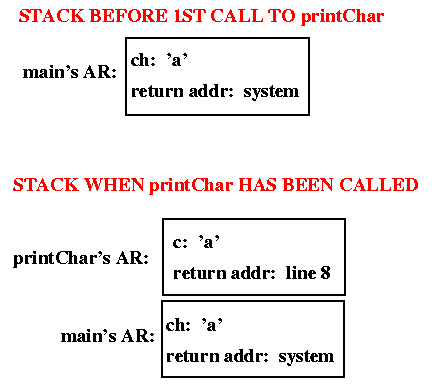When the first call to printChar returns, the top activation record is popped from the stack and the main method begins executing again at line 8. After executing the assignment at line 8, a second call to printChar is made (line 9), as illustrated below.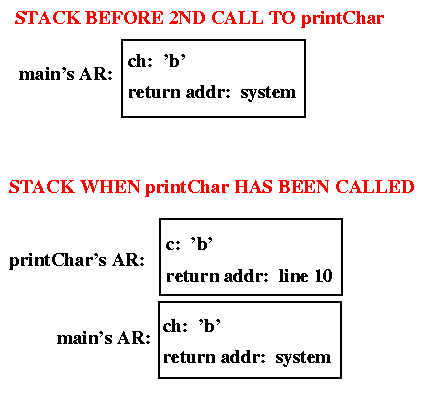The two calls to printChar return to different places in main because different return addresses are stored in the ARs that are pushed onto the stack when the calls are made.

Now let's consider recursive calls:

```1. void printInt( int k ) {
2.     if (k <= 0) return;
3.     System.out.println( k );
4.     printInt( k - 1 );
5. }
6.
7. void main(...) {
8.     printInt(2);
9.}
```
The following pictures illustrate the runtime stack as this program executes.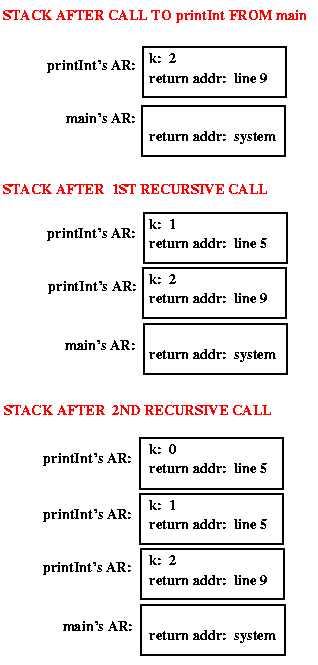Note that all of the recursive calls have the same return address -- after a recursive call returns, the previous call to printInt starts executing again at line 5. In this case, there is nothing more to do at that point, so the method would, in turn, return.

Note also that each call to printInt causes the current value of k to be printed, so the output of the program is: 2 1.

Now consider a slightly different version of the printInt method:

```1.  void printInt (int k) {
2.      if (k<=0) return;
3.      printInt (k-1);
4.      System.out.println(k)
5.  }
```
Now what is printed as a result of the call printInt(2)? Because the print statement comes after the recursive call, it is not executed until the recursive call finishes (i.e., printInt's activation record will have line 4 -- the print statement -- as its return address, so that line will be executed only after the recursive call finishes). In this case, that means that the output is: 1 2 (instead of 2 1, as it was when the print statement came before the recursive call). This leads to the following insight:

*** UNDERSTANDING RECURSION ***

Sometimes a method has more to do following a recursive call; it gets done only after the recursive call (and all calls it makes) are finished.

TEST YOURSELF #2

```void printTwoInts( int k ) {
if (k == 0) return;
System.out.println( "From before recursion: " + k );
printTwoInts( k - 1 );
System.out.println( "From after recursion: " + k );
}
```
What is printed as a result of the call printTwoInts(3)?

## Recursion vs Iteration

Now let's think about when it is a good idea to use recursion and why. In many cases there will be a choice: many methods can be written either with or without using recursion.

Q: Is the recursive version usually faster?
A: No -- it's usually slower (due to the overhead of maintaining the stack)

Q: Does the recursive version usually use less memory?
A: No -- it usually uses more memory (for the stack).

Q: Then why use recursion??
A: Sometimes it is much simpler to write the recursive version (but we'll need to wait until we've discussed trees to see really good examples...)

Here are a few examples where recursion makes things a little bit clearer, though in the second case, the efficiency problem makes it a bad choice.

### Factorial

Factorial can be defined as follows:

• factorial of 0 is 1
• factorial of N (for N>0) is N*N-1* ... *3*2*1
or:
• factorial of 0 is 1
• factorial of N (for N > 0) is N * factorial (N-1)
(Note that factorial is undefined for negative numbers.)

The first definition leads to an iterative version of factorial:

```int factorial (int N) {
if (N == 0) return 1;
int tmp = N;
for (int k=N-1; k>=1; k--) tmp = tmp*k;
return (tmp);
}
```
The second definition leads to a recursive version:
```int factorial (int N) {
if (N == 0) return 1;
else return (N*factorial(N-1));
}
```
The recursive version is
• a little shorter,
• a little clearer (closer to the mathematical definition),
• a little slower
Because the recursive version causes an activation record to be pushed onto the runtime stack for every call, it is also more limited than the iterative version (it will fail, with a "stack overflow" error), for large values of N.

TEST YOURSELF #3

Question 1:
Draw the runtime stack, showing the activation records that would be pushed as a result of the call factorial(3) (using the recursive version of factorial). Just show the value of N in each AR (don't worry about the return address). Also indicate the value that is returned as the result of each call.

Question 2:
Recall that (mathematically) factorial is undefined for a negative number. What happens as a result of the call factorial(-1) for each of the two versions (iterative and recursive)? What do you think really should happen when the call factorial(-1) is made? How could you write the method to make that happen?

### Fibonacci

Fibonacci can be defined as follows:

• fibonacci of 1 or 2 is 1
• fibonacci of N (for N>2) is fibonacci of (N-1) + fibonacci of (N-2)
Fibonacci can be programmed either using iteration, or using recursion. Here are the two versions (iterative first):
```// iterative version
int fib (int N) {
int k1, k2, k3;
k1 = k2 = k3 = 1;
for (int j = 3; j <= N; j++) {
k3 = k1 + k2;
k1 = k2;
k2 = k3;
}
return k3;
}

// recursive version
int fib (int N) {
if ((N == 1) || (N == 2)) return 1;
else return (fib (N-1) + fib (N-2));
}
```
For fibonacci, the recursive version is:
• shorter,
• clearer,
• but much slower
Here's a picture of a "trace" of the recursive version of fibonacci, for the initial call fib(6):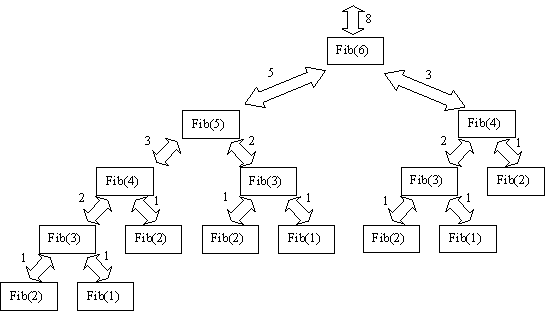Note: one reason the recursive version is so slow is that it is repeating computations. For example, fib(4) is computed twice, and fib(3) is computed 3 times.

Given that the recursive version of fibonacci is slower than the iterative version, is there a good reason for using it? The answer may be yes: because the recursive solution is so much simpler, it is likely to take much less time to write, debug, and maintain. If those costs (the cost for programming time) are more important than the cost of having a slow program, then the advantages of using the recursive solution outweigh the disadvantage, and you should use it! If the speed of the final code is of vital importance, then you should not use the recursive fibonacci.

## Using Mathematical Induction to Prove the Correctness of Recursive Code

If you like math, you may enjoy thinking about how to use mathematical induction to prove that recursive code is correct. If not, feel free to skip this section.

When using induction to prove a theorem, you need to show:

1. that the base case (usually n=0 or n=1) is true
2. that case k implies case k+1

It is sometimes straightforward to use induction to prove that recursive code is correct. Let's consider how to do that for the recursive version of factorial. First we need to prove the base case: factorial(0) = 0! (which, by definition is 1). The correctness of the factorial method for N=0 is obvious from the code: when N==0 it returns 1.

Now we need to show that if factorial(k) = k!, then factorial(k+1) = (k+1)!. Looking at the code we see that for N != 0, factorial(N) = (N)*factorial(N-1). So factorial(k+1) = (k+1)*factorial(k). By assumption, factorial(k) = k!, so factorial(k+1) = (k+1)*(k!). By definition, k! = k*(k-1)*(k-2)*...*3*2*1. So (k+1)*(k!) = (k+1)*k*(k-1)*(k-2)*...*3*2*1, which is (by definition) (k+1)!, and the proof is done.

Note that we've shown that the factorial method is correct for all values of N greater than or equal to zero (because our base case was N=0). We haven't shown anything about factorial for negative numbers.

## Summary

• Use recursion for clarity, and (sometimes) for a reduction in the time needed to write and debug code, not for space savings or speed of execution.
• Remember that every recursive method must have a base case (rule #1).
• Also remember that every recursive method must make progress towards its base case (rule #2).
• Sometimes a recursive method has more to do following a recursive call. It gets done only after the recursive call (and all calls it makes) finishes.
• Recursion is often simple and elegant, can be efficient, and tends to be underutilized. Consider using recursion when solving a problem!